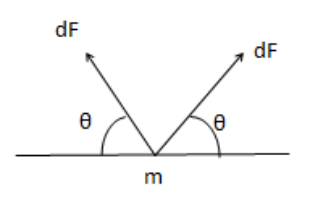# Solve the following :`
Question:

Suppose the rod with the balls $A$ and $B$ of the previous problem is clamped at the centre in such a way that it can rotate freely about a horizontal axis through the clamp. The system is kept at rest in the horizontal position. A particle $P$ of the same mass $m$ is dropped from a height $h$ on the ball $B$. The particle collides with B and sticks to it. (a) Find the angular momentum and the angular speed of the system just after the collision. (b) What should be the minimum value of $h$ so that the system makes a full rotation after the collision.

Solution:(a) If we consider two bodies $P$ and $B$ as system

then $F_{\text {ext }}=0$ and ${ }^{\tau_{\text {ext }}}=0$

$P_{i}=P_{f}$

$\mathrm{m}(\sqrt{2 g h})=2 m v^{\prime}$

$V^{\prime}=\sqrt{\frac{g h}{2}}$.

$L_{i}=L_{f}$

$\mathrm{m}(\sqrt{2 g h})\left(\frac{L}{2}\right)=\mid \omega$

$m L \sqrt{\frac{g h}{2}}=\left(\frac{2 m L^{2}}{4}+\frac{m L^{2}}{4}\right) \omega$

$\omega=\frac{\sqrt{8 g h}}{3 L}$